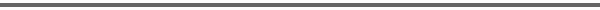Journal Home Page Complete Contentsof this Volume Previous Article Next Article Journal of Lie Theory 14 (2004), No. 1, 011--023 Copyright Heldermann Verlag 2004On the Nilpotency of Certain Subalgebras of Kac-Moody Lie Algebras Yeonok Kim Dept. of Mathematics, Soong Sil University, Seoul 151, South Korea, yokim@ssu.ac.kr Kailash C. Misra, Ernie Stitzinger Dept. of Mathematics, North Carolina State University, Raleigh, NC 27695-8205, USA, misra@math.ncsu.edu, stitz@math.ncsu.edu[Abstract-pdf] Let $\g = \n_-\oplus\h\oplus\n_+$ be an indecomposable Kac-Moody Lie algebra associated with the generalized Cartan matrix $A=(a_{ij})$ and $W$ be its Weyl group. For $w \in W$, we study the nilpotency index of the subalgebra $S_w =\n_+ \cap w(\n_-)$ and find that it is bounded by a constant $k=k(A)$ which depends only on $A$ but not on $w$ for all $A=(a_{ij})$ finite, affine of type other than $E$ or $F$ and indefinite type with $|a_{ij}| \geq 2$. In each case we find the best possible bound $k$. In the case when $A=(a_{ij})$ is hyperbolic of rank two we show that the nilpotency index is either 1 or 2. [ Fulltext-pdf  (197  KB)] for subscribers only.